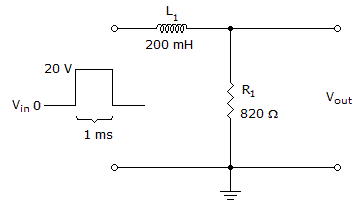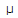# Electronics - Time Response of Reactive Circuits - Discussion

### Discussion :: Time Response of Reactive Circuits - Filling the Blanks (Q.No.2)

2.It takes ______ for the output voltage in the given circuit to reach its maximum value.

 [A]. 0 s [B]. 243.9s [C]. 1 ms [D]. 1.22 ms

Explanation:

No answer description available for this question.

 Kiran V said: (Dec 22, 2016) Time constant for RL circuit = T. T = L/R, T = (200 * 10^-3)/(820), T = 0.2439 ms. For the output voltage in the given circuit to reach its maximum value requires = 5 * T. ie.,(5 * Time constant). 5 * T = 5 * 0.2439 * 10^-3 = 1.2195 ms.

 Abhinak said: (Jan 19, 2018) How @Kiran V?